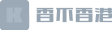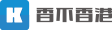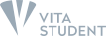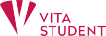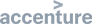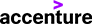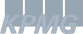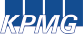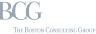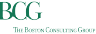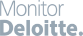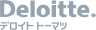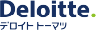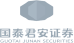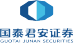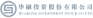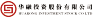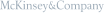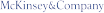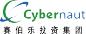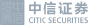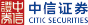Master of Science in Mathematics   院校：新加坡国立大学   学院：理学院

1年

50新加坡元

37,150新加坡元

Curriculum
• 交换代数
Commutative Algebra
• 数理逻辑
Mathematical Logic
• 偏微分方程式
Partial Differential Equations
• 代数拓扑
Algebraic Topology
• 逻辑和数学基础
Logic and Foundation of Mathematics
• 微分几何
Differential Geometry
• 计算数学
Computational Mathematics
• 建模和数值模拟
Modeling and Numerical Simulations
• 有限元法
Finite Element Method
• 运筹学专题研究
关于留学的一切都在这里

### 联系我们

内地总部
深圳市福田区皇岗路5001号深业上城T2写字楼2907
400-632-8005
香港总部
香港荃湾杨屋道8号如心广场19楼
+852 62302196最新资讯 留学干货一手掌握
合作伙伴